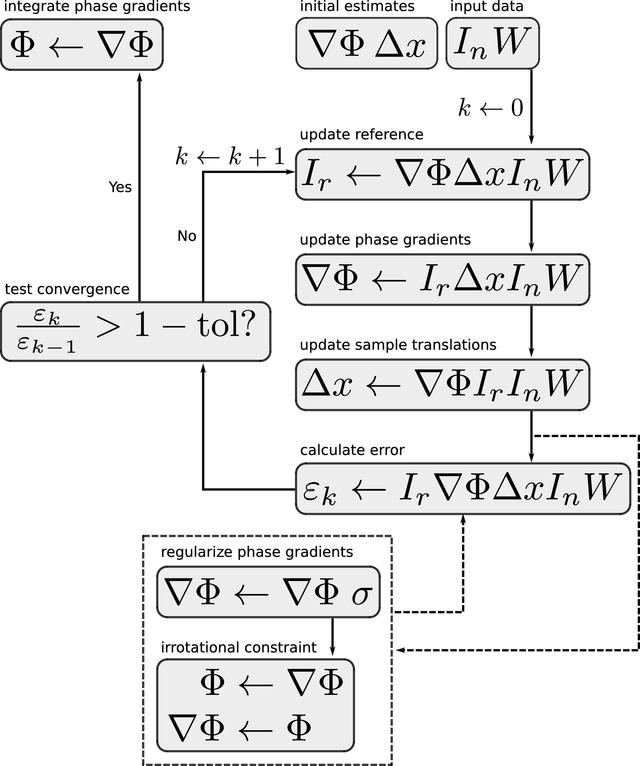disable zoom     view article Figure 6 Flow diagram for the PXST iterative refinement cycle. A left arrow (←) represents an update of the item on the left given the items to the right of the arrow. The dashed line arrows represent optional paths in the algorithm. Each step in the diagram corresponds to an equation in the main text: `update reference' to equation (27), `update phase gradients' to equation (28), `update sample translation' to equation (29), `calculate error' to equation (26), `regularize phase gradients' to equation (30), and finally the `irrotational constraint' and `integrate phase gradients' steps to equation (31).JOURNAL OFAPPLIEDCRYSTALLOGRAPHY
ISSN: 1600-5767
Volume 53| Part 3| June 2020| Pages 760-780